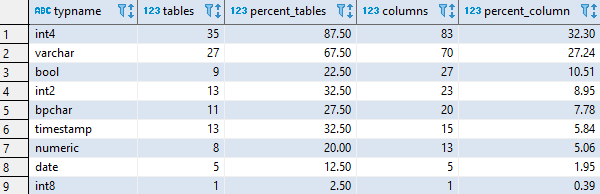## Find most used data type in Redshift

Article for:

Query below returns data types used in a database ordered by the number of their occurance.

## Query

``````with stats as (
select count(distinct col.table_schema||'.'||col.table_name)
as sum_tables,
count(col.column_name) as sum_columns
from information_schema.columns col
join information_schema.tables tab
on col.table_schema = tab.table_schema
and col.table_name = tab.table_name
where tab.table_schema not in ('information_schema', 'pg_catalog')
and tab.table_type = 'BASE TABLE'
)
select col.udt_name as typname,
count(distinct col.table_schema||'.'||col.table_name) as tables,
round(100.0*
count(distinct col.table_schema||'.'||col.table_name)
/ (select sum_tables from stats)
, 2) as percent_tables,
count(col.column_name) as columns,
round(100.0*count(col.column_name)
/ (select sum_columns from stats)
, 2) as percent_column
from information_schema.columns col
join information_schema.tables tab
on col.table_schema = tab.table_schema
and col.table_name = tab.table_name
where tab.table_schema not in ('information_schema', 'pg_catalog')
and tab.table_type = 'BASE TABLE'
group by col.udt_name
order by columns desc,
tables desc;
``````

## Columns

• typname - built in or user data type without length or precision, e.g. int, varchar or date
• tables - number of tables in a database with this data type
• percent_tables - percentage of tables with columns with this data type.
• columns - number of columns in a database with this data type
• percent_columns - percentage of columns with this data type. Rows add up to 100%.

## Rows

• One row represents one data type
• Scope of rows: all data types used in a database
• Ordered by number of columns descending, number of tables descending

## Sample results